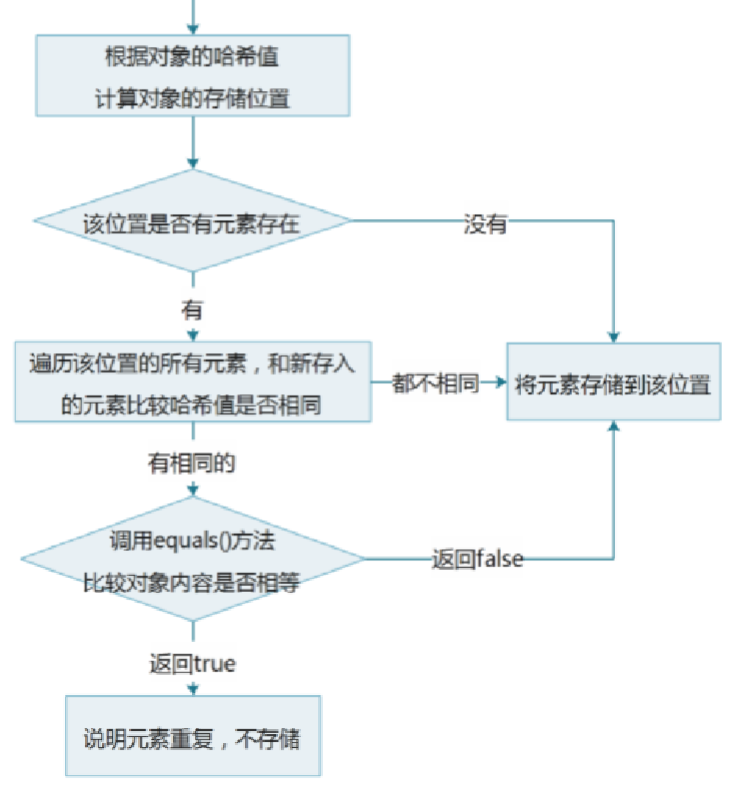# HashSet集合保证元素唯一性的源码分析

##### 源码

public boolean add(E e) {
}

###### 进入put()方法，在put方法中我们可以发现，返回了一个putVal()方法，首先我们看到方法中的第一个参数hash(key)，下方提供①hash()方法帮助理解，接着我们不难发现，我们添加的元素e在此处变成了key，而且hash()方法已经算出了元素的哈希值key
public V put(K key, V value) {
return putVal(hash(key), key, value, false, true);
}

final V putVal(int hash, K key, V value, boolean onlyIfAbsent,
boolean evict) {
Node<K,V>[] tab; Node<K,V> p; int n, i;
//如果哈希表没有初始化，就对其进行初始化操作
if ((tab = table) == null || (n = tab.length) == 0)
n = (tab = resize()).length;
//根据元素的哈希值计算存储位置，如果这个位置上没有元素，就把元素存储进去
if ((p = tab[i = (n - 1) & hash]) == null)
tab[i] = newNode(hash, key, value, null);
else {
Node<K,V> e; K k;
/*如果该位置上有元素，就把我们要存入的元素的哈希值和这个元素比较：
情况一：哈希值不同，便会向下执行，存储元素
情况二：哈希值相同，调用元素的equals()方法比较元素：
情况一：元素不同，向下执行，存储元素
情况二：元素相同，停止执行，不存储*/
if (p.hash == hash &&
((k = p.key) == key || (key != null && key.equals(k))))
e = p;
.......//省略部分代码
}

###### ①hash()方法，其实hash()方法就是一个计算哈希值的方法，key为计算好的哈希值
static final int hash(Object key) {
int h;
return (key == null) ? 0 : (h = key.hashCode()) ^ (h >>> 16);
}


### 总结